Olympiad Test : Mensuration

# Olympiad Test : Mensuration

Test Description

## 20 Questions MCQ Test Maths Olympiad Class 6 | Olympiad Test : Mensuration

Olympiad Test : Mensuration for Class 6 2022 is part of Maths Olympiad Class 6 preparation. The Olympiad Test : Mensuration questions and answers have been prepared according to the Class 6 exam syllabus.The Olympiad Test : Mensuration MCQs are made for Class 6 2022 Exam. Find important definitions, questions, notes, meanings, examples, exercises, MCQs and online tests for Olympiad Test : Mensuration below.
Solutions of Olympiad Test : Mensuration questions in English are available as part of our Maths Olympiad Class 6 for Class 6 & Olympiad Test : Mensuration solutions in Hindi for Maths Olympiad Class 6 course. Download more important topics, notes, lectures and mock test series for Class 6 Exam by signing up for free. Attempt Olympiad Test : Mensuration | 20 questions in 40 minutes | Mock test for Class 6 preparation | Free important questions MCQ to study Maths Olympiad Class 6 for Class 6 Exam | Download free PDF with solutions
 1 Crore+ students have signed up on EduRev. Have you?
Olympiad Test : Mensuration - Question 1

### If a wire of length 267 cm be bent to form an equilateral triangle, then what is the length of side of the equilateral triangle?

Detailed Solution for Olympiad Test : Mensuration - Question 1

If x is required length then
x + x + x = 267
3x = 267 ⇒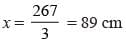Olympiad Test : Mensuration - Question 2

### Mohit runs a distance of 7km 800m in going round a rectangular ground three times. What is the length of the ground if its width is 330m?

Detailed Solution for Olympiad Test : Mensuration - Question 2

Here, 3 × perimeter of rectangular ground = 7800 m
⇒ 3 [2 (l + b)] = 7800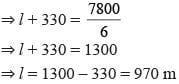Olympiad Test : Mensuration - Question 3

### What is the perimeter of an isosceles triangles with equal sides 8.5cm each and third side 7cm?

Detailed Solution for Olympiad Test : Mensuration - Question 3

Perimeter of isosceles triangle
= 8.5 + 8.5 + 7 = 24 cm

Olympiad Test : Mensuration - Question 4

What is the perimeter of regular hexagon having each side 6.5 cm?

Detailed Solution for Olympiad Test : Mensuration - Question 4

Perimeter of regular hexagon
= 6 × 6.5 cm = 39 cm

Olympiad Test : Mensuration - Question 5

The cost of fencing a rectangular field at Rs 18 per metre is Rs 1980. If the width of the field is 23m, what is its length?

Detailed Solution for Olympiad Test : Mensuration - Question 5

Here, Perimeter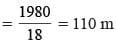If l  and b are its length and breadth, then
⇒ 2(l + b) = 110
⇒ 2(l + 23) = 110
⇒ l + 23 = 55 ⇒ l = 55 - 23 = 32m

Olympiad Test : Mensuration - Question 6

What is the diameter of the circle whose circumference is 66 cm?

Detailed Solution for Olympiad Test : Mensuration - Question 6

Circumference of circle = 66
2πr = 66 where r is radius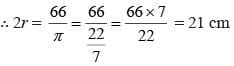Olympiad Test : Mensuration - Question 7

What is the radius of the circle whose circumference is 264 cm?

Detailed Solution for Olympiad Test : Mensuration - Question 7

Given,
2π r = 264 where r is radius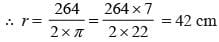Olympiad Test : Mensuration - Question 8

The diameter of the wheel of a car is 70 cm. How many revolutions will it make to travel 1.65km?

Detailed Solution for Olympiad Test : Mensuration - Question 8

Circumference of wheel of car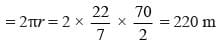∴ Required no. of revolutions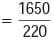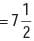Olympiad Test : Mensuration - Question 9

What is the circumference of a circle whose diameter is 35cm?

Detailed Solution for Olympiad Test : Mensuration - Question 9

Circumference of circle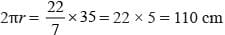Olympiad Test : Mensuration - Question 10

The area of a rectangle is 630 cm2 and its length is 35cm, what is its perimeter?

Detailed Solution for Olympiad Test : Mensuration - Question 10

If b  is breadth of rectangle then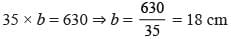Perimeter = 2 (l + b) = 2 (35 + 18)
= 2 × 53 = 106 cm

Olympiad Test : Mensuration - Question 11

The total cost of flooring a room at Rs 85/m2 is Rs 5100. If the length of the room is 8m, then what is its width?

Detailed Solution for Olympiad Test : Mensuration - Question 11

Area of the room =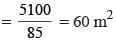If b  is its width then
l × b = 60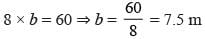Olympiad Test : Mensuration - Question 12

The area of a rectangle is 540 cm2. Its length is 36cm, what is its perimeter?

Detailed Solution for Olympiad Test : Mensuration - Question 12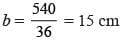∴ Perimeter of rectangle = 2 ( 36 + 15)
= 2 × 51 = 102 cm.

Olympiad Test : Mensuration - Question 13

What is the area of square whose diagonal is 5√2 cm?

Detailed Solution for Olympiad Test : Mensuration - Question 13

Area of square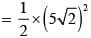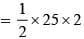= 25 cm2

Olympiad Test : Mensuration - Question 14

The length and breadth of a rectangular park are in the ratio 5 : 3 and its perimeter is 128m. What is the area of the park?

Detailed Solution for Olympiad Test : Mensuration - Question 14

If 5x and 3x are length and breadth then
2 (5x + 3x) = 128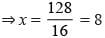∴ l = 5 × 8 = 40 m
and b = 3 × 8 = 24 m
Hence, area = l × b = 40 × 24 = 960 m2

Olympiad Test : Mensuration - Question 15

What is the number of square tiles of size 10cm required for decorating a wall of 12 m × 8 m?

Detailed Solution for Olympiad Test : Mensuration - Question 15

Required no. of square tiles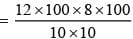= 9600

Olympiad Test : Mensuration - Question 16

Five flower beds each of side 3cm are dug on a piece of land 17m long and 5m wide. What is the area of remaining part of the land?

Detailed Solution for Olympiad Test : Mensuration - Question 16

Area of one flower bed = 3 × 3 = 9 cm2
Area of five flower beds = 9 × 5 = 45 cm2
Area of rectangular field = 17 × 5    = 85 cm2
Area of remaining part = 85 – 45 = 40 cm2

Olympiad Test : Mensuration - Question 17

The perimeter of the given figure is 37 cm. find the missing value.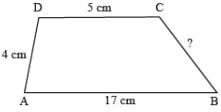Detailed Solution for Olympiad Test : Mensuration - Question 17

Missing value = 37 – (5 + 4 + 17)
= 37 – 26 = 11 cm

Olympiad Test : Mensuration - Question 18

The area of a square is 2401 cm2. What is its perimeter?

Detailed Solution for Olympiad Test : Mensuration - Question 18

Area of square = 2401 = 49 × 49
∴ Side of square = 49 cm
Hence, perimeter of square
= 4 × side = 4 × 49 = 196 cm

Olympiad Test : Mensuration - Question 19

The diagonal of a square is 8√2 cm what is its perimeter?

Detailed Solution for Olympiad Test : Mensuration - Question 19

Area of square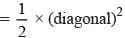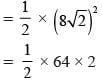= 64
∴ Side of square = 8 cm
Hence, perimeter of square
= 4 × 8 = 32 cm

Olympiad Test : Mensuration - Question 20

A floor is 7m long and 5m wide. A square carpet of sides 4m is laid on the floor. What is the area of floor that is not carpeted?

Detailed Solution for Olympiad Test : Mensuration - Question 20

Area of floor = 7 × 5 = 35 m2
Area of carpet = 4 × 4 = 16 m2
Remaining part = 35 – 16 = 19 m2

## Maths Olympiad Class 6

43 tests
 Use Code STAYHOME200 and get INR 200 additional OFF Use Coupon Code
Information about Olympiad Test : Mensuration Page
In this test you can find the Exam questions for Olympiad Test : Mensuration solved & explained in the simplest way possible. Besides giving Questions and answers for Olympiad Test : Mensuration , EduRev gives you an ample number of Online tests for practice

43 tests